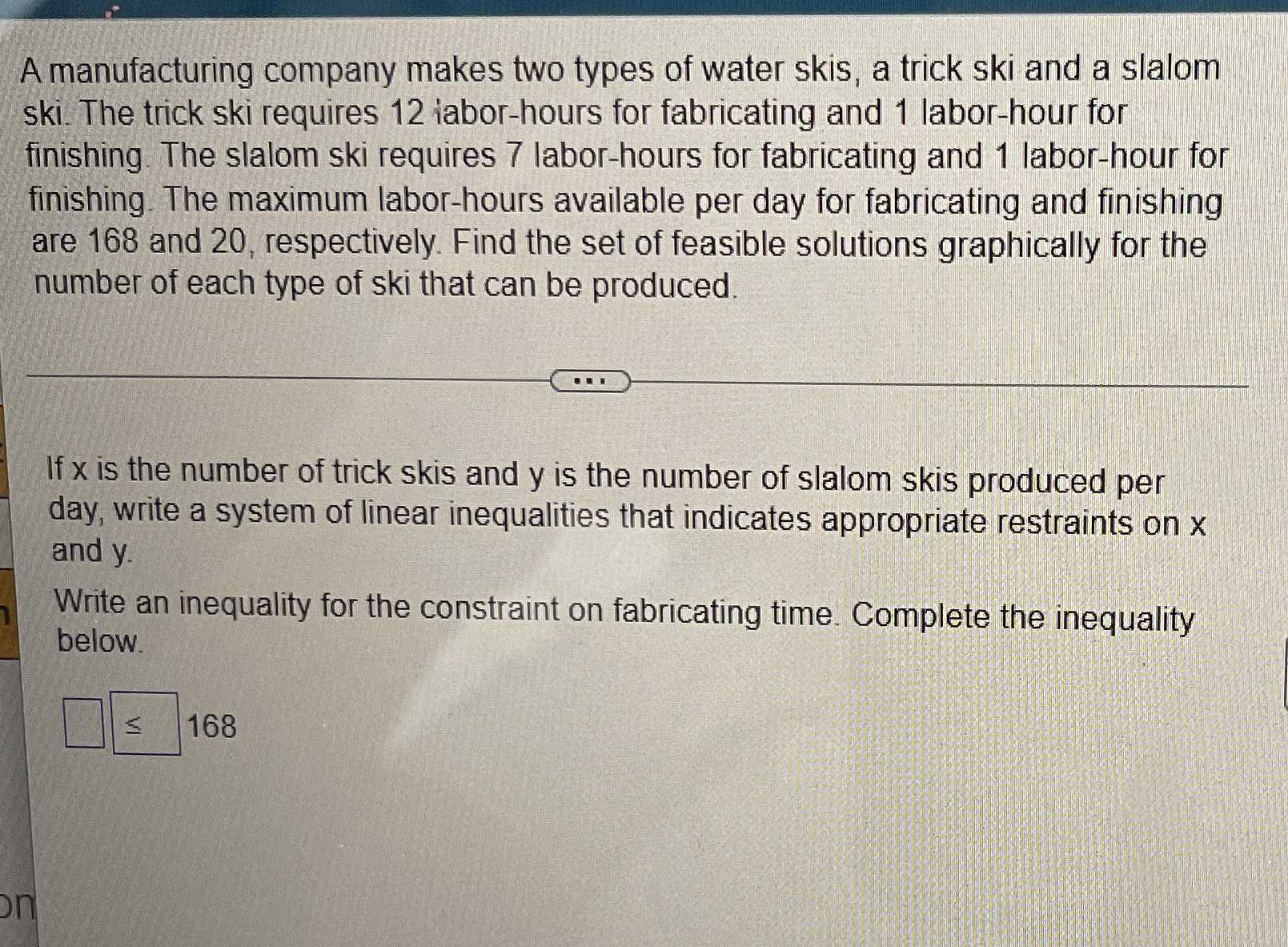### Still have math questions?

Algebra
QuestionA manufacturing company makes two types of water skis, a trick ski and a slalom ski. The trick ski requires $$12$$ iabor-hours for fabricating and $$1$$ labor-hour for finishing. The slalom ski requires $$7$$ labor-hours for fabricating and $$1$$ labor-hour for finishing. The maximum labor-hours available per day for fabricating and finishing are $$168$$ and $$20$$ , respectively. Find the set of feasible solutions graphically for the number of each type of ski that can be produced.

If $$x$$ is the number of trick skis and y is the number of slalom skis produced per day, write a system of linear inequalities that indicates appropriate restraints on $$x$$ and y.

Write an inequality for the constraint on fabricating time. Complete the inequality below.

$$12x+ 7y\le 168$$
$$x+ y\le 20$$